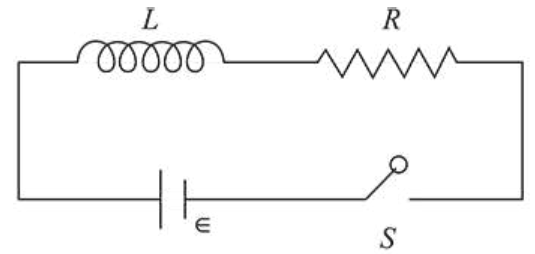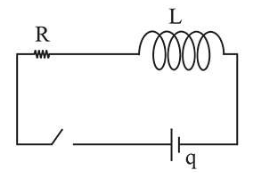# As shown in the figure, a battery of emf in is connected to an inductor L and resistance R in series.

Question:As shown in the figure, a battery of emf $\in$ is connected to an inductor $L$ and resistance $R$ in series. The switch is closed at $t=0$. The total charge that flows from the battery, between $t=0$ and $t=t_{c}\left(t_{c}\right.$ is the time constant of the circuit) is:

1. (1) $\frac{\in}{e L}$

2. (2) $\frac{\in L}{R^{2}}\left(1-\frac{1}{e}\right)$

3. (3) $\frac{\in L}{R^{2}}$

4. (4) $\frac{\in R}{e L^{2}}$

Correct Option: 1,

Solution:

(1)

For series connection of a resistor and inductor, time

variation of current is $I=I_{0}\left(1-e^{-t / T_{c}}\right)$Here, $T_{C}=\frac{L}{R}$

$q=\int^{T_{c}} i d t$

$\Rightarrow \int d q=\int \frac{E}{R}\left(1-e^{-t / t_{c}}\right) d t$

$\Rightarrow q=\frac{\in}{R}\left[t+t_{C} e^{-t / t_{c}}\right]_{0}^{t_{c}}$

$\Rightarrow q=\frac{\in}{R}\left[t_{C}+\frac{t_{C}}{e}-t_{C}\right]$

$\Rightarrow q=\frac{\epsilon}{R} \frac{L}{\operatorname{Re}}$

$\therefore q=\frac{\in L}{R^{2} e}$